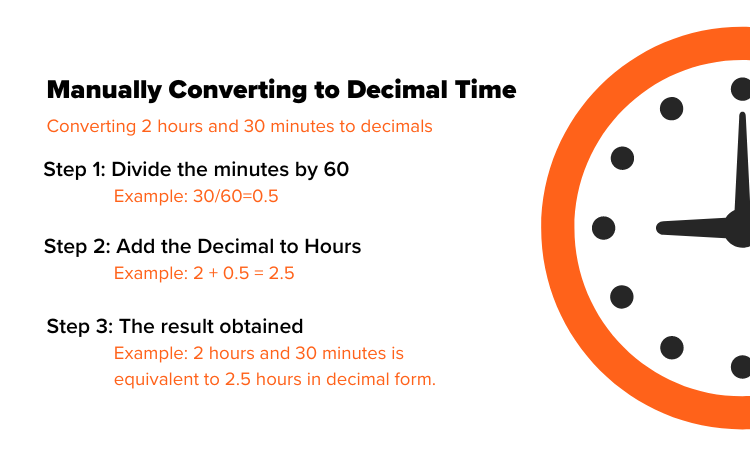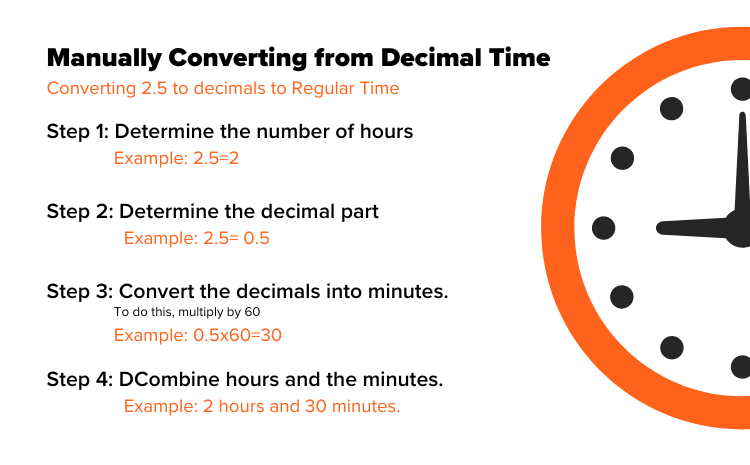# Decimal Hours Calculator

Convert hours and minutes to decimal hours with our simple online calculator.

=

## Convert Time to a Decimal Format Instantly

Decimal hours are a common way to calculate the total time worked. They’re best for determining an hourly salary or the total time spent on a project. You can use our calculator to instantly convert hours and minutes to decimal hours to speed up your timesheet process.

Converting from hours and minutes to decimals can be a hassle, especially if you're dealing with large amounts of data or need to convert times frequently. If that's your situation, then OnTheClock's time-tracking software can make the process much easier.

OnTheClock simplifies the time-conversion process by eliminating the need to convert times manually. Our time clock has the ability to display time worked in decimals or hours and minutes — one of our many customizable features designed to meet your needs.

Our software also integrates with payroll providers like Quickbooks, Gusto, ADP, and more. This will save you even more time by calculating gross and net pay, as well as PTO, overtime, holidays, and more. These highly accurate timesheets will help you streamline your payroll process while staying compliant with labor regulations and company policies.

Your first 30 days of OnTheClock are free — .

### Decimal Chart

Here’s a handy reference you can use to convert decimals to and from hours and minutes. To use this decimal conversion chart, locate your starting number in the “Minutes” or “Decimal” column. Then, match it with the decimal hour or minutes in the column next to it.

For example, if you want to convert 45 minutes into decimals, locate “45” in the table (under the “Minutes” column) and then follow the row to the corresponding decimal (in the “Decimals” column). In this case, 45 minutes is equal to 0.75 decimal hours.

In addition, you can also use the table to convert from decimals back into minutes and hours, by simply finding the decimal number in the “Decimal” column and then following it across to the left.

Minutes Decimal Minutes Decimal Minutes Decimal
1 .01 21 .35 41 .68
2 .03 22 .37 42 .70
3 .05 23 .38 43 .72
4 .07 24 .40 44 .73
5 .08 25 .42 45 .75
6 .10 26 .43 46 .77
7 .12 27 .45 47 .78
8 .13 28 .47 48 .50
9 .15 29 .48 49 .82
10 .17 30 .50 50 .83
11 .18 31 .52 51 .85
12 .20 32 .53 52 .87
13 .22 33 .55 53 .88
14 .23 34 .57 54 .90
15 .25 35 .58 55 .92
16 .27 36 .60 56 .93
17 .28 37 .62 57 .95
18 .30 38 .63 58 .97
19 .32 39 .65 59 .98
20 .33 40 .67 60 1.0

## Manual Conversion

Prefer a mental challenge? Here’s how to do the math behind decimal time conversion

### Manually Converting to Decimal Time

Step 1: Divide the minutes by 60 to convert them into decimal form.

Example: If you have 30 minutes, divide 30 by 60: 30/60 = 0.5

Step 2: Add the decimal form of the minutes to the hours.

Example: If you have 2 hours and 30 minutes, add 2 (hours) and 0.5 (decimal form of minutes): 2 + 0.5 = 2.5

Step 3: The result obtained in step 2 represents the time duration in decimal format. The whole number part before the decimal represents the hours, and the decimal part represents the fraction of an hour (in this case, the minutes).

So, 2 hours and 30 minutes is equivalent to 2.5 hours in decimal form.### Manually Converting from Decimal Time

Step 1: Determine the whole number of hours.

Example: If you have 2.5 hours, the whole number of hours is 2.

Step 2: Determine the decimal part as a fraction of an hour.

Example: In 2.5 hours, the decimal part is 0.5, which represents 0.5 of an hour.

Step 3: Convert the decimal part into minutes. To do this, multiply by 60.

Example: 0.5 multiplied by 60 = 30 minutes.

Step 4: Combine the whole number of hours and the minutes. Example: 2 hours and 30 minutes.

So, 2.5 hours is equivalent to 2 hours and 30 minutes.

A

There are a few ways to calculate total working hours in a decimal format:

• Use a decimal hours calculator
• Use a decimal hours conversion chart
• Manually convert minutes to decimals by dividing by 60
• Use time clock software to automatically calculate

Automatically converting to decimal format with time clock software is the easiest, since there is no additional work required.

A

To calculate payroll with a decimal hours calculator, simply enter the total time worked into the calculator to generate a number with a decimal. Then, multiply the decimal hour by your hourly wage rate. Their result will be their gross pay.

So, for example, say you’re calculating the paycheck for someone who worked 78 hours and 45 minutes during a 2-week pay period. Their hours worked would be 78.75 hours in decimal format. If their hourly pay rate is \$18 an hour, you’d multiply 78.75 by 18. This will produce their gross pay for the given period, which is \$1,417.50.

From there, you’ll need to manually calculate deductions and withholdings based on your local regulations and company policies. Using time clock software that integrates with payroll software will automatically calculate this for you, creating accurate payroll in just a few clicks.

A

For payroll, it’s much easier to calculate a pay rate with decimal hours rather than hours and minutes. Additionally, decimal hours are much more precise.

### Time duration calculator

Calculate the duration between two times instantly.